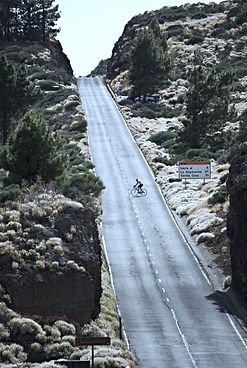## Tour de France 2014

###### 15 Jul 2014

If the data of Vincenzo Nibali's dominating performance in Stage 5 of this year's TdF is correct (cyclingpro.it), then it is interesting to make some considerations on the numbers reported.

3h19’ at 285w average correspond to about 3600 Kcal.

In the stage, Nibali rode approximately:

- 75' at 180w average = 800 Kcal

- 34' at 260w average = 530 Kcal

- 26' at 320w average = 510 Kcal

- 24' to 390W average = 560 Kcal

- 17' at 450w average = 460 kcal

- 16' to 530W medium = 500 Kcal

- 8' above 600w = 290 kcal

Of the above caloric expenditure, 65' (from/above 390W average) were obtained, given the intensity, by CHO alone for a total of 1810 Kcal = 450g of glycogen.

Another 60' (from 260w to 320w average) came from the mix of approximately 70% CHO - 30% fat, with an additional consumption of 180g of glycogen.

The remaining 75' at 180w average utilized a mix of  50% CHO - 50% fat, which means another 100g of glycogen burned.

A total of 730g of glycogen assume a supersaturation of muscle and liver deposits that is difficult to achieve for an athlete of 63kg, within the few hours between two stages of the TdF.

Hence the hypothesis that Nibali actually expressed an incredibly high LIPID POWER that has allowed him to consume less glycogen than calculated above.

From the the average power outputs that he expressed (41 min above 450w) we can imagine an AT4 of at least 440W, equal to 6.98 w/kg, assuming a weight of 63 kg.